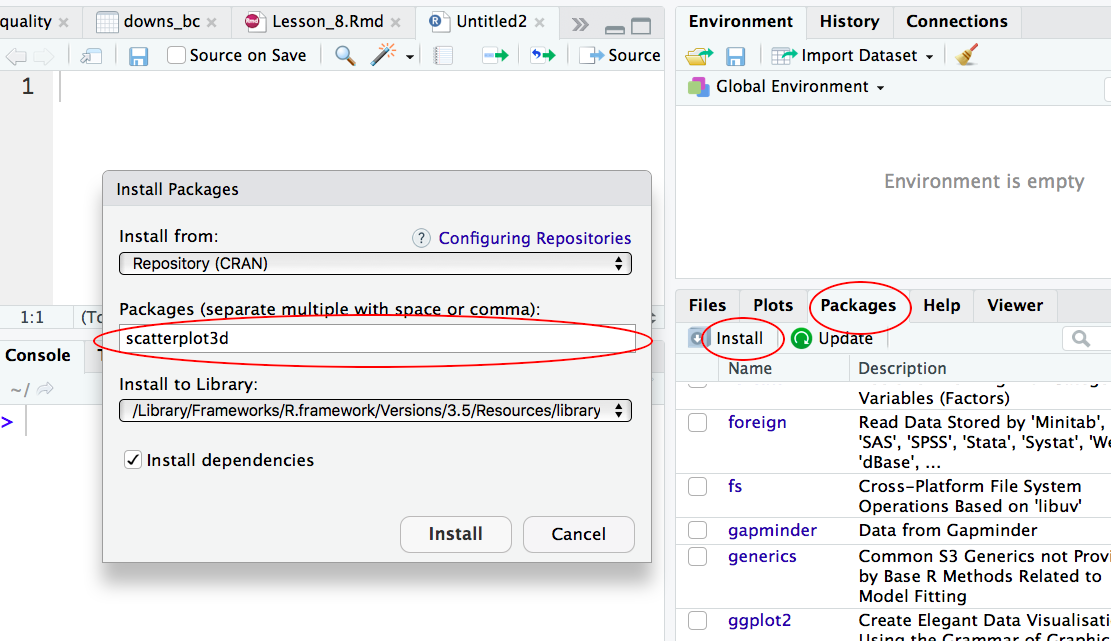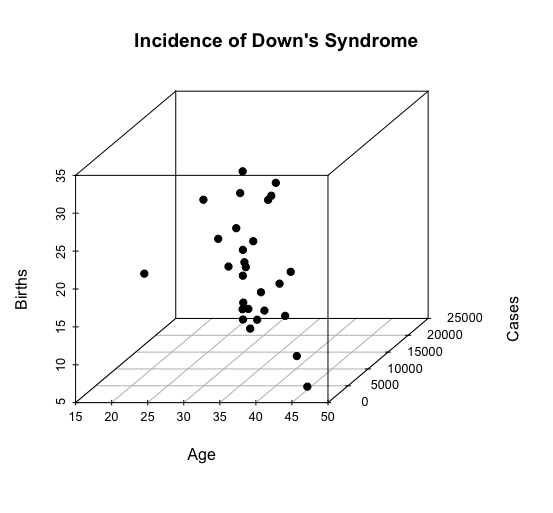# Lesson 8 — 3D scatterplot

#### By Olivia Mah

First published on March 16, 2019

### Learning Objectives

Create 3D scatterplots

### Demonstration

Here is the dataset that we are using in this demo:

downs_bc.csv

This dataset is about Down’s Syndrome in Canada. (I have slightly modified the original dataset for our purpose).

https://vincentarelbundock.github.io/Rdatasets/doc/boot/downs.bc.html

We will first import the dataset. Then we will generate a 3D scatterplot from the dataset downs_bc. See below for details.

#### Step 1: Install the R package “scatterplot3d”

Two ways to install a R package one way is to type install.packages(scatterplot3d); the other way is to install via the graphical interface of RStudio:#### Step 2: Install the R package “scatterplot3d”

Before we can use the R package to create 3D scatterplots, we need to load the package into R first:

> library(scatterplot3d)

#### Step 3: Create a 3D scatterplot

Our dataset is called downs_bc. We want to generate a 3D scatterplot for the three columns in that dataset: cases, age and births.

> library(scatterplot3d)
> with(downs_bc,
+      scatterplot3d(cases ~ age + births, pch = 19,
+                    main="Incidence of Down's Syndrome",
+                    xlab="Age", ylab="Cases", zlab="Births"))



Explanation:

We use the library function to load the R package “scatterplot3d“.

Then we use generate the 3D scatterplot from the databset downs_bc:

cases ~ age means that the variable cases is explained by age (i.e. age is the explanatory variable);

+ births means that the variable births is the third variable in this scatterplot;

pch = 19 means to use plotting symbol 19 (solid circle) in our plot. (Type ?pch at the prompt to find out more about pch.)

Here is the 3D scatterplot:-END-